The OpenFOAM Foundation
SpatialVector< Cmpt > Class Template Reference

Templated 3D spatial vector derived from VectorSpace used to represent the anglular and linear components of position, velocity and acceleration of rigid bodies. More...

Inheritance diagram for SpatialVector< Cmpt >: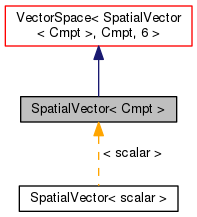[legend]
Collaboration diagram for SpatialVector< Cmpt >: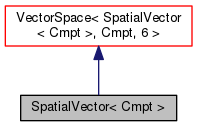[legend]

## Classes

class  dual
Class to represent the dual spatial vector. More...

## Public Types

enum  components {
WX, WY, WZ, LX,
LY, LZ
}
Component labeling enumeration. More...Public Types inherited from VectorSpace< SpatialVector< Cmpt >, Cmpt, 6 >
typedef VectorSpace< SpatialVector< Cmpt >, Cmpt, Ncmpts > vsType
VectorSpace type. More...

typedef Cmpt cmptType
Component type. More...

## Public Member Functions

SpatialVector ()
Construct null. More...

SpatialVector (const Foam::zero)
Construct initialized to zero. More...

SpatialVector (const typename SpatialVector::vsType &)
Construct given VectorSpace of the same rank. More...

SpatialVector (const Vector< Cmpt > &w, const Vector< Cmpt > &l)
Construct from the angular and linear vector components. More...

SpatialVector (const Cmpt &wx, const Cmpt &wy, const Cmpt &wz, const Cmpt &lx, const Cmpt &ly, const Cmpt &lz)
Construct given 6 components. More...

SpatialVector (Istream &)
Construct from Istream. More...

const Cmpt & wx () const

const Cmpt & wy () const

const Cmpt & wz () const

const Cmpt & lx () const

const Cmpt & ly () const

const Cmpt & lz () const

Cmpt & wx ()

Cmpt & wy ()

Cmpt & wz ()

Cmpt & lx ()

Cmpt & ly ()

Cmpt & lz ()

Vector< Cmpt > w () const
Return the angular part of the spatial vector as a vector. More...

Vector< Cmpt > l () const
Return the linear part of the spatial vector as a vector. More...

dual operator* () const
Return the dual spatial vector. More...Public Member Functions inherited from VectorSpace< SpatialVector< Cmpt >, Cmpt, 6 >
VectorSpace ()
Construct null. More...

VectorSpace (const Foam::zero)
Construct initialized to zero. More...

VectorSpace (Istream &)
Construct from Istream. More...

VectorSpace (const VectorSpace< SpatialVector< Cmpt >, Cmpt, Ncmpts > &)
Construct as copy. More...

VectorSpace (const VectorSpace< Form2, Cmpt2, Ncmpts > &)
Construct as copy of a VectorSpace with the same size. More...

const Cmpt & component (const direction) const

Cmpt & component (const direction)

void component (Cmpt &, const direction) const

void replace (const direction, const Cmpt &)

const ConstBlock< SubVector, BStart > block () const

const Cmpt & operator[] (const direction) const

Cmpt & operator[] (const direction)

void operator= (const VectorSpace< SpatialVector< Cmpt >, Cmpt, Ncmpts > &)

void operator= (const Foam::zero)

void operator+= (const VectorSpace< SpatialVector< Cmpt >, Cmpt, Ncmpts > &)

void operator-= (const VectorSpace< SpatialVector< Cmpt >, Cmpt, Ncmpts > &)

void operator*= (const scalar)

void operator/= (const scalar)Static Public Member Functions inherited from VectorSpace< SpatialVector< Cmpt >, Cmpt, 6 >
static direction size ()
Return the number of elements in the VectorSpace = Ncmpts. More...

static SpatialVector< Cmpt > uniform (const Cmpt &s)
Return a VectorSpace with all elements = s. More...Public Attributes inherited from VectorSpace< SpatialVector< Cmpt >, Cmpt, 6 >
Cmpt v_ [Ncmpts]
The components of this vector space. More...Static Public Attributes inherited from VectorSpace< SpatialVector< Cmpt >, Cmpt, 6 >
static const direction dim
Dimensionality of space. More...

static const direction nComponents
Number of components in this vector space. More...

static const direction mRows

static const direction nCols

static const char *const typeName

static const char *const componentNames []

static const SpatialVector< Cmpt > zero

static const SpatialVector< Cmpt > one

static const SpatialVector< Cmpt > max

static const SpatialVector< Cmpt > min

static const SpatialVector< Cmpt > rootMax

static const SpatialVector< Cmpt > rootMin

## Detailed Description

### template<class Cmpt> class Foam::SpatialVector< Cmpt >

Templated 3D spatial vector derived from VectorSpace used to represent the anglular and linear components of position, velocity and acceleration of rigid bodies.

Reference:

```    Featherstone, R. (2008).
Rigid body dynamics algorithms.
Springer.
```
Source files
Foam::VectorSpace Foam::Vector

Definition at line 62 of file SpatialVector.H.

## Member Enumeration Documentation

 enum components

Component labeling enumeration.

Enumerator
WX
WY
WZ
LX
LY
LZ

Definition at line 70 of file SpatialVector.H.

## Constructor & Destructor Documentation

 SpatialVector ( )
inline

Construct null.

Definition at line 29 of file SpatialVectorI.H.

Referenced by SpatialVector< Cmpt >::SpatialVector().

Here is the caller graph for this function: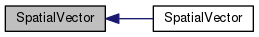SpatialVector ( const Foam::zero )
inline

Construct initialized to zero.

Definition at line 34 of file SpatialVectorI.H.

References SpatialVector< Cmpt >::SpatialVector().

Here is the call graph for this function: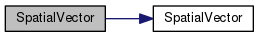SpatialVector ( const typename SpatialVector< Cmpt >::vsType & vs )
inline

Construct given VectorSpace of the same rank.

Definition at line 42 of file SpatialVectorI.H.

References SpatialVector< Cmpt >::SpatialVector().

Here is the call graph for this function:SpatialVector ( const Vector< Cmpt > & w, const Vector< Cmpt > & l )
inline

Construct from the angular and linear vector components.

Definition at line 52 of file SpatialVectorI.H.

Here is the call graph for this function: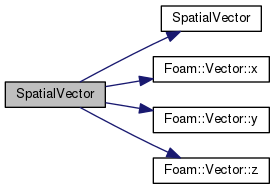SpatialVector ( const Cmpt & wx, const Cmpt & wy, const Cmpt & wz, const Cmpt & lx, const Cmpt & ly, const Cmpt & lz )
inline

Construct given 6 components.

Definition at line 68 of file SpatialVectorI.H.

 SpatialVector ( Istream & is )
inline

Construct from Istream.

Definition at line 87 of file SpatialVectorI.H.

## Member Function Documentation

 const Cmpt & wx ( ) const
inline

Definition at line 103 of file SpatialVectorI.H.

References SpatialVector< Cmpt >::WX.

Referenced by Rx::jcalc(), and Foam::operator^().

Here is the caller graph for this function: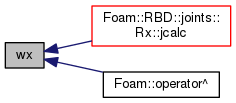const Cmpt & wy ( ) const
inline

Definition at line 110 of file SpatialVectorI.H.

References SpatialVector< Cmpt >::WY.

Referenced by Ry::jcalc(), and Foam::operator^().

Here is the caller graph for this function: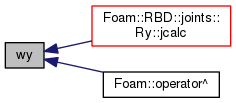const Cmpt & wz ( ) const
inline

Definition at line 117 of file SpatialVectorI.H.

References SpatialVector< Cmpt >::WZ.

Referenced by Rz::jcalc(), and Foam::operator^().

Here is the caller graph for this function: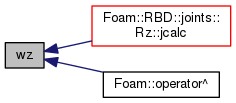const Cmpt & lx ( ) const
inline

Definition at line 124 of file SpatialVectorI.H.

References SpatialVector< Cmpt >::LX.

Referenced by Foam::operator^().

Here is the caller graph for this function: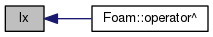const Cmpt & ly ( ) const
inline

Definition at line 131 of file SpatialVectorI.H.

References SpatialVector< Cmpt >::LY.

Referenced by Foam::operator^().

Here is the caller graph for this function: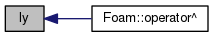const Cmpt & lz ( ) const
inline

Definition at line 138 of file SpatialVectorI.H.

References SpatialVector< Cmpt >::LZ.

Referenced by Foam::operator^().

Here is the caller graph for this function: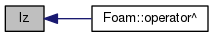Cmpt & wx ( )
inline

Definition at line 145 of file SpatialVectorI.H.

References SpatialVector< Cmpt >::WX.

 Cmpt & wy ( )
inline

Definition at line 152 of file SpatialVectorI.H.

References SpatialVector< Cmpt >::WY.

 Cmpt & wz ( )
inline

Definition at line 159 of file SpatialVectorI.H.

References SpatialVector< Cmpt >::WZ.

 Cmpt & lx ( )
inline

Definition at line 166 of file SpatialVectorI.H.

References SpatialVector< Cmpt >::LX.

 Cmpt & ly ( )
inline

Definition at line 173 of file SpatialVectorI.H.

References SpatialVector< Cmpt >::LY.

 Cmpt & lz ( )
inline

Definition at line 180 of file SpatialVectorI.H.

References SpatialVector< Cmpt >::LZ.

 Foam::Vector< Cmpt > w ( ) const
inline

Return the angular part of the spatial vector as a vector.

Definition at line 187 of file SpatialVectorI.H.

Referenced by rigidBodyMotion::status().

Here is the caller graph for this function: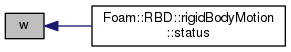Foam::Vector< Cmpt > l ( ) const
inline

Return the linear part of the spatial vector as a vector.

Definition at line 193 of file SpatialVectorI.H.

Referenced by rigidBodyMotion::status().

Here is the caller graph for this function: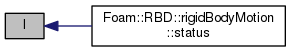Foam::SpatialVector< Cmpt >::dual operator* ( ) const
inline

Return the dual spatial vector.

Definition at line 210 of file SpatialVectorI.H.

References SpatialVector< Cmpt >::dual::dual().

Here is the call graph for this function: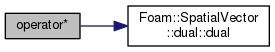The documentation for this class was generated from the following files: